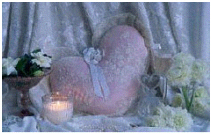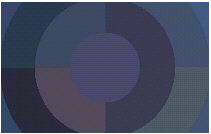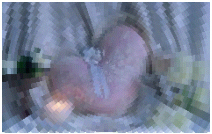﻿ PixelateCommand Class | Leadtools.ImageProcessing.SpecialEffects | Raster, Medical, Document Help
←Select platform
In This Topic ▼

# PixelateCommand Class

Summary

Divides the image into rectangular or circular cells and then recreates the image by filling those cells with the minimum, maximum, or average pixel value, depending upon the effect that was selected. This command is available in the Imaging Pro and above toolkits.

Syntax
C#
VB
C++
``public class PixelateCommand : RasterCommand ``
````  `
`Public Class PixelateCommand  `
`   Inherits RasterCommand ````
``public ref class PixelateCommand : public RasterCommand   ``
Remarks
• This command will divide the image into rectangular or circular cells.
• Flags indicate whether to use rectangular or circular cells and indicates the type of information in the other properties or parameters.
• If the image is divided into circular cells by setting the PixelateCommandFlags.Radial flag, the cells will be centered around the specified center point. This center point must be defined inside the image or inside the region, if the image has a region. If the image has a region, the effect will be applied on the region only.
• This command supports 12-bit and 16-bit grayscale and 48-bit and 64-bit color images. Support for 12-bit and 16-bit grayscale and 48-bit and 64-bit color images is available only in the Document/Medical toolkits.
• An example of circular cell division can be seen below: This is the original image:The image below is the result of the following settings: command.Flags = PixelateCommandFlags.Radial | PixelateCommandFlags.WidthPeriod | PixelateCommandFlags.HeightPeriod | PixelateCommandFlags.Average; command.CellWidth = 90; command.CellHeight = 40; This indicates the circular cells are divided into 90 degree cell divisions and each cell has a radial length of 40 pixels. Each cell division is filled with the average value for that cell division.The image below is the result of the following settings: command.Flags = PixelateCommandFlags.Radial | PixelateCommandFlags.WidthFrequency | PixelateCommandFlags.HeightFrequency | PixelateCommandFlags.Average; command.CellWidth = 90; command.CellHeight = 40; This indicates the circular cells are divided into 90 separate cell divisions around the center point and there are 40 cell divisions along the radius. Each cell division is filled with the average value for that cell division.• This command does not support signed data images.

• This command does not support 32-bit grayscale images.

Example

Run the PixelateCommand on an image. by dividing it into circular cells with angle length = 5, and radius = 10.

C#
VB
````using Leadtools; `
`using Leadtools.Codecs; `
`using Leadtools.ImageProcessing.SpecialEffects; `
` `
`public void PixelateCommandExample() `
`{ `
`   // Load an image `
`   RasterCodecs codecs = new RasterCodecs(); `
`   codecs.ThrowExceptionsOnInvalidImages = true; `
` `
`   RasterImage image = codecs.Load(Path.Combine(LEAD_VARS.ImagesDir, "sample5.cmp")); `
` `
`   // Prepare the command `
`   PixelateCommand command = new PixelateCommand(); `
`   command.CenterPoint = new LeadPoint((image.Width / 2), (image.Height / 2)); `
`   command.CellWidth = 5; `
`   command.CellHeight = 10; `
`   command.Opacity = 100; `
`   command.Flags = PixelateCommandFlags.Radial | `
`      PixelateCommandFlags.WidthPeriod | `
`      PixelateCommandFlags.HeightPeriod | `
`      PixelateCommandFlags.Average; `
`   command.Run(image); `
`   codecs.Save(image, Path.Combine(LEAD_VARS.ImagesDir, "Result.jpg"), RasterImageFormat.Jpeg, 24); `
` `
`} `
` `
`static class LEAD_VARS `
`{ `
`   public const string ImagesDir = @"C:\Users\Public\Documents\LEADTOOLS Images"; `
`} ````
````Imports Leadtools `
`Imports Leadtools.Codecs `
`Imports Leadtools.ImageProcessing.SpecialEffects `
` `
`Public Sub PixelateCommandExample() `
`   Dim codecs As New RasterCodecs() `
`   codecs.ThrowExceptionsOnInvalidImages = True `
` `
`   Dim leadImage As RasterImage = codecs.Load(Path.Combine(LEAD_VARS.ImagesDir, "sample5.cmp")) `
` `
`   ' Prepare the command `
`   Dim command As PixelateCommand = New PixelateCommand `
`   command.CenterPoint = New LeadPoint(leadImage.Width \ 2, leadImage.Height \ 2) `
`   command.CellWidth = 5 `
`   command.CellHeight = 10 `
`   command.Opacity = 100 `
`   command.Flags = PixelateCommandFlags.Radial Or `
`      PixelateCommandFlags.WidthPeriod Or `
`      PixelateCommandFlags.HeightPeriod Or `
`   PixelateCommandFlags.Average `
`   command.Run(leadImage) `
`   codecs.Save(leadImage, Path.Combine(LEAD_VARS.ImagesDir, "Result.jpg"), RasterImageFormat.Jpeg, 24) `
` `
`End Sub `
` `
`Public NotInheritable Class LEAD_VARS `
`   Public Const ImagesDir As String = "C:\Users\Public\Documents\LEADTOOLS Images" `
`End Class ````
Requirements
Help Version 20.0.2019.11.25